2.7. Orthogonal series estimators

Orthogonal series estimators approach the density estimation problem from quite a different point of view. They are best explained by a specific example. Suppose that we are trying to estimate a density f on the unit interval [0, 1]. The idea of the orthogonal series method is then to estimate f by estimating the coefficients of its Fourier expansion.

Define the sequence(x) by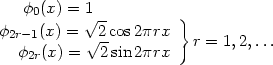Then, by standard mathematical analysis, f can be represented as the Fourier series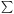=0f, where, for each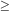0,(2.6)

For a discussion of the sense in which f is represented by the series, see, for example, Kreider et al. (1966).

Suppose X is a random variable with density f. Then (2.6) can be written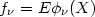and hence a natural, and unbiased, estimator of fbased on a sample X1,..., Xn from f is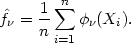Unfortunately, the sum=0will not be a good estimate of f, but will `converge' to a sum of delta functions at the observations; to see this, let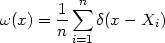(2.7)

whereis the Dirac delta function. Then, for each,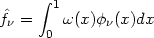and so theare exactly the Fourier coefficients of the function.

In order to obtain a useful estimate of the density f, it is necessary to smoothby applying a low-pass filter to the sequence of coefficients. The easiest way to do this is to truncate the expansionat some point. Choose an integer K and define the density estimateby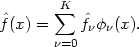(2.8)

The choice of the cutoff point K determines the amount of smoothing.

A more general approach is to taper the series by a sequence of weights, which satisfy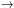0 as, to obtain the estimate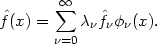The rate at which the weightsconverge to zero will determine the amount of smoothing.

Other orthogonal series estimates, no longer necessarily confined to data lying on a finite interval, can be obtained by using different orthonormal sequences of functions. Suppose a(x) is a weighting function and () is a series satisfying, for µ and0,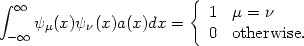For instance, for data resealed to have zero mean and unit variance, a(x) might be the function e-x2/2 and themultiples of the Hermite polynomials; for details see Kreider et al. (1966).

The sample coefficients will then be defined by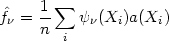but otherwise the estimates will be defined as above; possible estimates are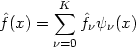(2.9)

or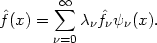(2.10)

The properties of estimates obtained by the orthogonal series method depend on the details of the series being used and on the system of weights. The Fourier series estimates will integrate to unity, provided0 = 1, since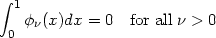and0 will always be equal to one. However, except for rather special choices of the weights,cannot be guaranteed to be non-negative. The local smoothness properties of the estimates will again depend on the particular case; estimates obtained from (2.8) will have derivatives of all orders.